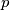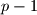### IMO Shortlist 2003 problem N8

Kvaliteta:
Avg: 0,0
Težina:
Avg: 9,0
Let$p$ be a prime number and let$A$ be a set of positive integers that satisfies the following conditions: (1) the set of prime divisors of the elements in$A$ consists of$p-1$ elements; (2) for any nonempty subset of$A$, the product of its elements is not a perfect$p$-th power. What is the largest possible number of elements in$A$ ?
Izvor: Međunarodna matematička olimpijada, shortlist 2003